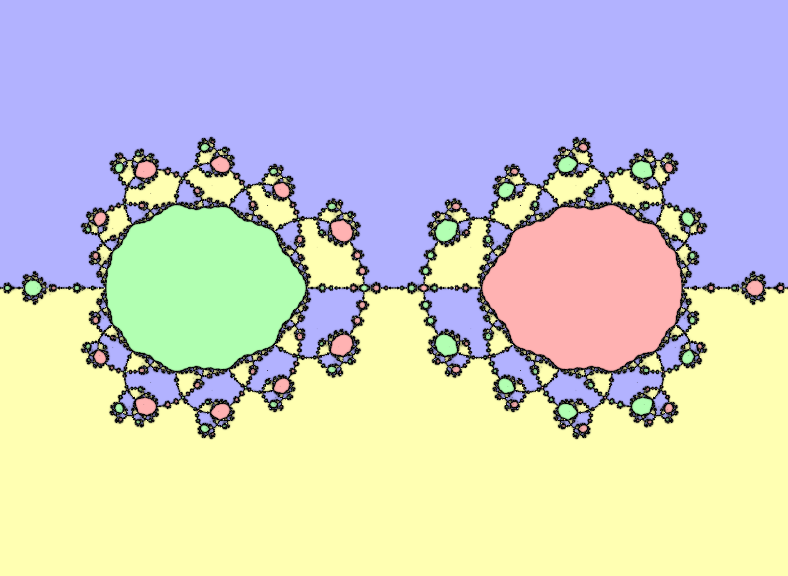# mathr / blog / #

## Distance estimation for Newton fractalsI started with distance estimator for Julia sets for the case of a super-attracting fixed point:

$\delta = - \lim_{k \to \infty} \frac{|z_k - z_\infty| \log |z_k - z_\infty|}{|\frac{d}{dz}_k|}$

This formula is slightly different to the formula on the linked page, haven't worked out yet exactly why it works and what the significance of the differences are. Anyway, I wanted to apply it to Newton fractals for rational functions.

Recall Newton's root finding method for a function $$G(z)$$:

$z_{k+1} = z_k - \frac{G(z_k)}{\frac{d}{dz}G(z_k)}$

If there are more than two roots of G, the boundary between regions that converge to different roots is a fractal. It's actually a Julia set for $$F(z)$$ where

$F(z) = z - \frac{G(z)}{\frac{d}{dz}G(z)}$

So we need to compute $$F^k(z)$$ and$$\frac{d}{dz}F^k(z)$$ for the distance estimate. By the product rule for derivatives, the derivative is the product of the derivatives at each step. It turns out that the actual calculations are very simple. Here's the derivation:

\begin{aligned} F(z) &= z - \frac{G(z)}{\frac{d}{dz}G(z)} \\ \frac{d}{dz} F(z) &= 1 - (\frac{d}{dz} G(z) \frac{1}{\frac{d}{dz} G(z)} + G(z) \frac{d}{dz} \frac{1}{\frac{d}{dz} G(z)}) \\ &= 1 - (1 + G(z) \frac{- \frac{d}{dz}\frac{d}{dz} G(z)}{(\frac{d}{dz} G(z))^2})\\ &= \frac{G(z) \frac{d}{dz}\frac{d}{dz} G(z)}{(\frac{d}{dz} G(z))^2} \end{aligned}

As $$\frac{d}{dz}F(z)$$ has a factor $$G(z)$$, and iterations of F(z) converge to a root $$z_\infty$$ where $$G(z_\infty) = 0$$, the roots are super-attracting fixed points.

Now, $$G(z)$$ is a rational function:

$G(z) = \frac{P(z)}{Q(z)} = \frac{\prod_{i} (z - p_i)^{P_i}}{\prod_{j} (z - q_j)^{Q_j}}$

We need to compute $$F$$ and $$\frac{d}{dz}F$$, and happily this doesn't need the calculation of all of $$G$$, $$\frac{d}{dz}G$$ and $$\frac{d}{dz}\frac{d}{dz}G$$, because lots of terms cancel each other out:

\begin{aligned} \frac{d}{dz} G(z) &= \frac{ Q(z) \frac{d}{dz} P(z) - P(z) \frac{d}{dz} Q(z) }{ (Q(z))^2 } \\ \frac{d}{dz} P(z) &= \sum_I{(P_I (z - p_I)^{P_I - 1} \prod_{i \ne I} (z - p_i)^P_i)} \\ &= (\prod_i{ (z-p_i)^P_i }) (\sum_i{ \frac{P_i}{z - p_i}}) \\ &= (\sum_i{ \frac{P_i}{z - p_i}}) P(z) \\ \frac{d}{dz} Q(z) &= (\sum_j{ \frac{Q_j}{z - q_j}}) Q(z) \\ \frac{G(z)}{\frac{d}{dz}G(z)} &= \frac{\frac{P(z)}{Q(z)}}{\frac{Q(z)(\sum_i{ \frac{P_i}{z - p_i}})P(z) - P(z) (\sum_j{ \frac{Q_j}{z - q_j}}) Q(z)}{(Q(z))^2}}) \\ &= (P / Q) / ((P Q (\sum_P) - P Q (\sum_Q)) / (Q Q)) \\ &= 1 / ((\sum_P) - (\sum_Q)) \\ F(z) &= z - \frac{1}{(\sum_i{ \frac{P_i}{z - p_i} }) - (\sum_j{ \frac{Q_j}{z - q_j}})} \\ \frac{d}{dz} F(z) &= 1 + \frac{ (\sum_j{ \frac{Q_j}{(z - q_j)^2}}) - (\sum_i{ \frac{P_i}{(z - p_i)^2} }) }{((\sum_i{ \frac{P_i}{z - p_i} }) - (\sum_j{ \frac{Q_j}{z - q_j}}))^2} \end{aligned}

where the derivation of the last line is left as an exercise (in other words, I couldn't be bothered to type up all the pages of equations I scribbled on paper).

Putting it into code, here's the algorithm in C99:

#include <complex.h>
#include <math.h>

typedef unsigned int N;
typedef double R;
typedef double complex C;

R                 // OUTPUT the distance estimate
distance
( C z0            // INPUT  starting point
, N nzero         // INPUT  number of zeros
, const C *zero   // INPUT  the zeros
, const C *zerop  // INPUT  the power of each zero
, N npole         // INPUT  number of poles
, const C *pole   // INPUT  the poles
, const C *polep  // INPUT  the power of each pole
, N *which        // OUTPUT the index of the zero converged to
) {
C z = z0;
C dz = 1.0;
R eps = 0.1;    // root radius, should be as large as possible
for (N k = 0; k < 1024; ++k) { // fixed iteration limit
for (N i = 0; i < nzero; ++i) { // check if converged
R e = cabs(z - zero[i]);
if (e < eps) {
*which = i;
return e * -log(e) / cabs(dz); // compute distance
}
}
C sz = 0.0;
C sz2 = 0.0;
for (N i = 0; i < nzero; ++i) {
C d = z - zero[i];
sz += zerop[i] / d;
sz2 += zerop[i] / (d * d);
}
C sp = 0.0;
C sp2 = 0.0;
for (N j = 0; j < npole; ++j) {
C d = z - pole[j];
sp += polep[j] / d;
sp2 += polep[j] / (d * d);
}
C d = sz - sp;
z -= 1.0 / d;
dz *= (sp2 - sz2) / (d * d) + 1.0;
}
*which = nzero;
return -1;  // didn't converge
}